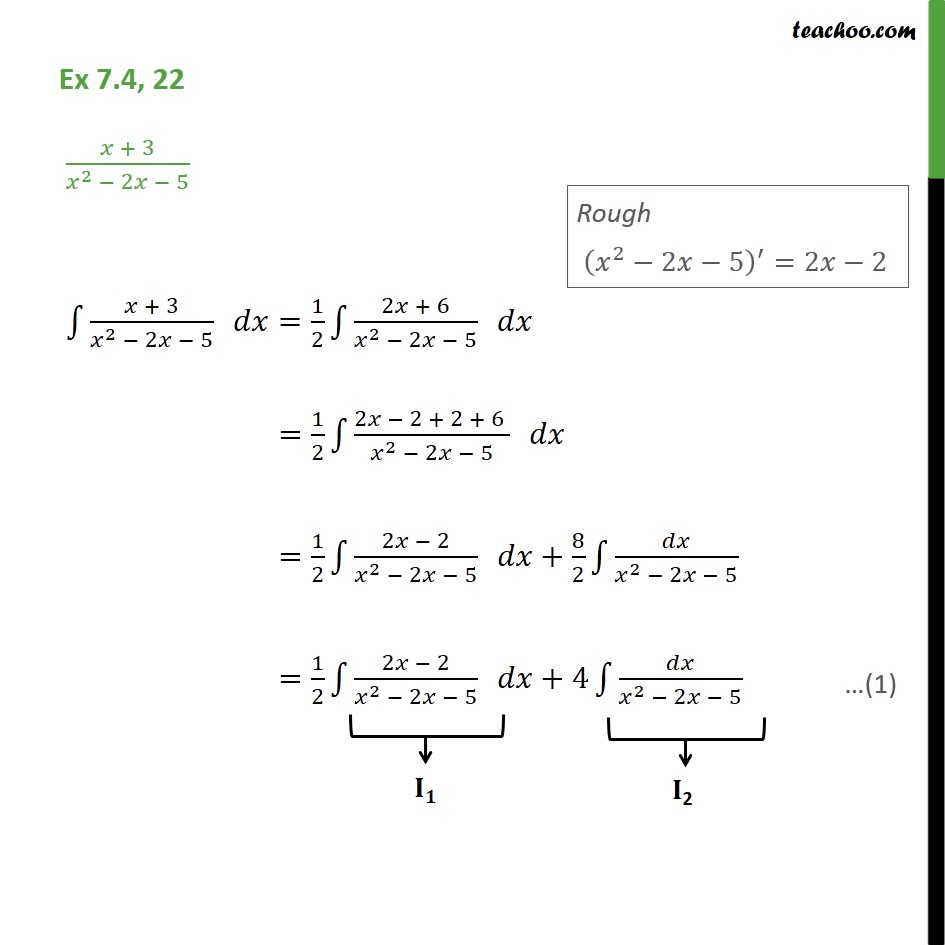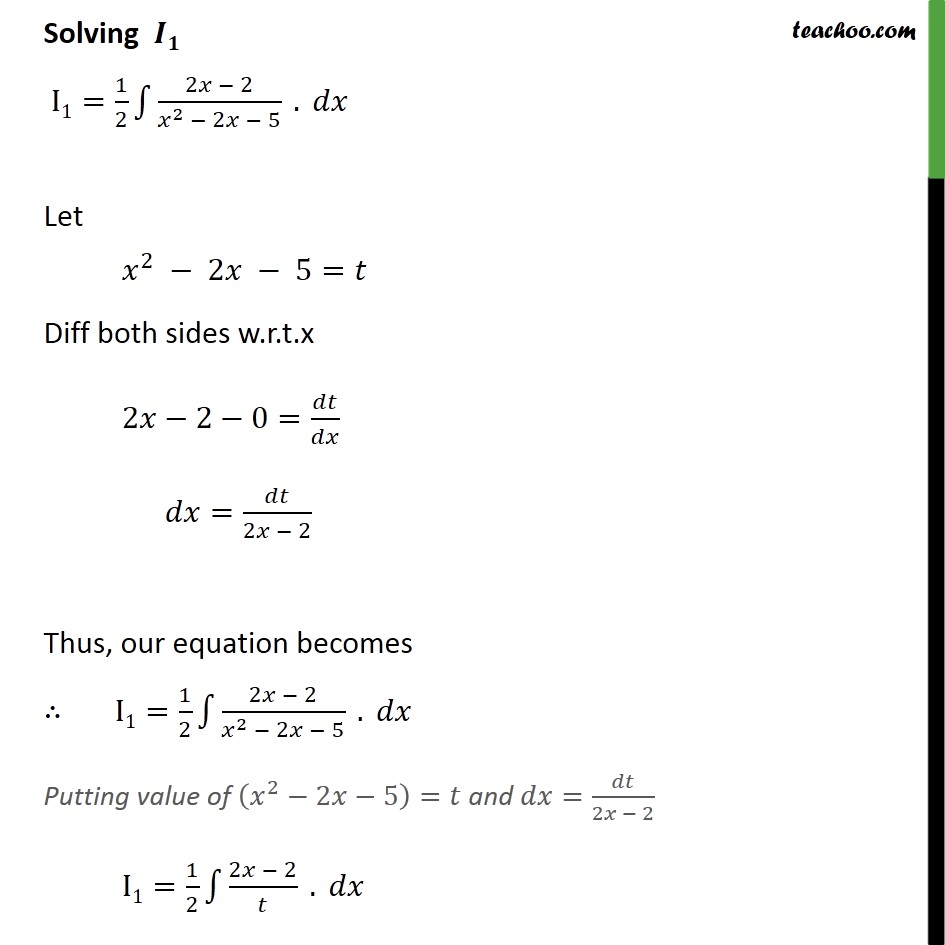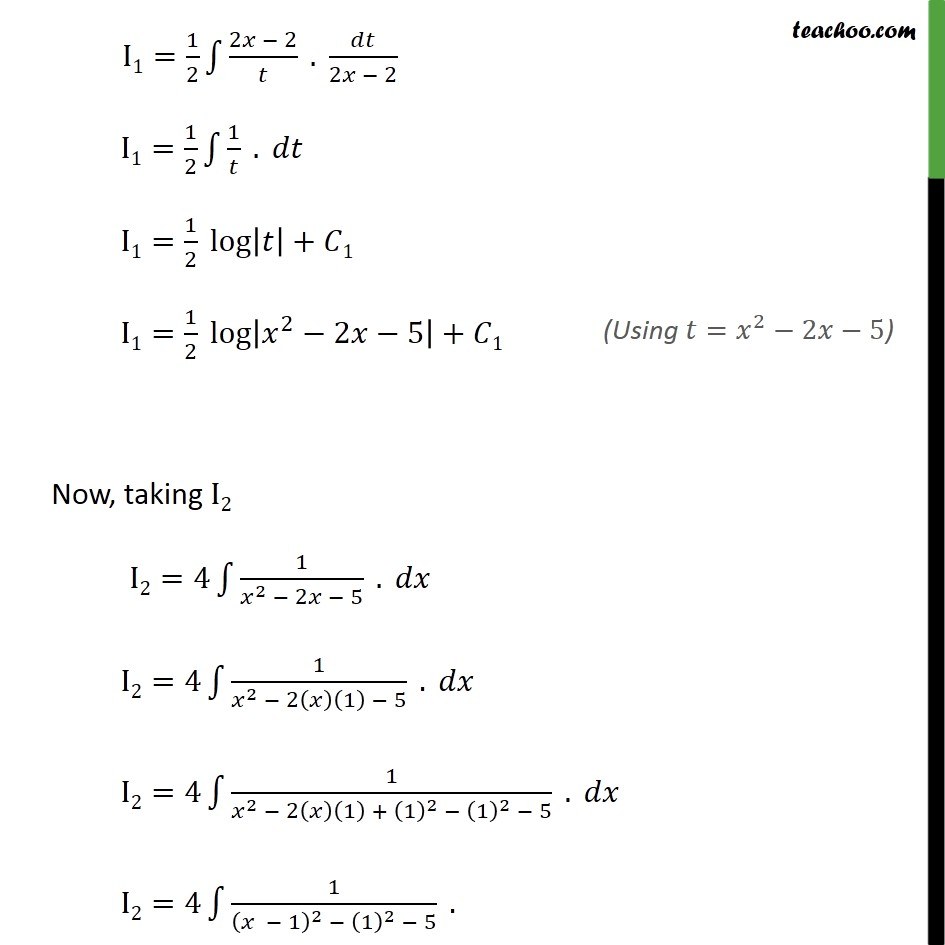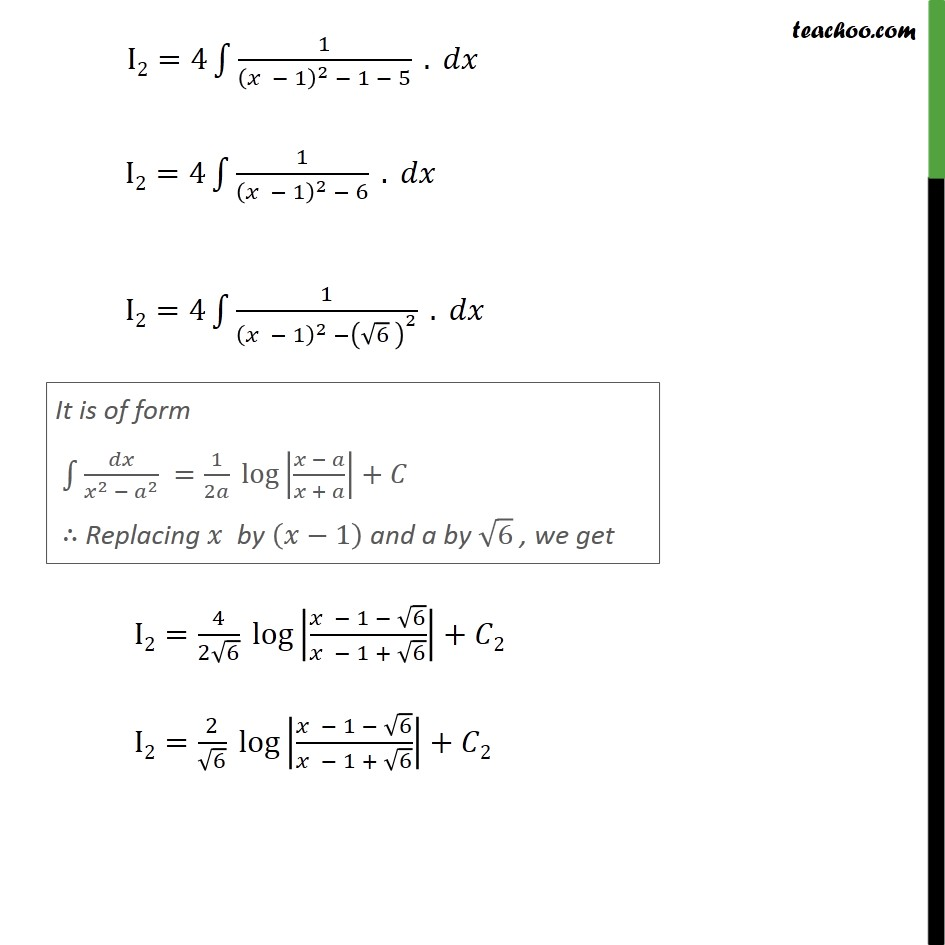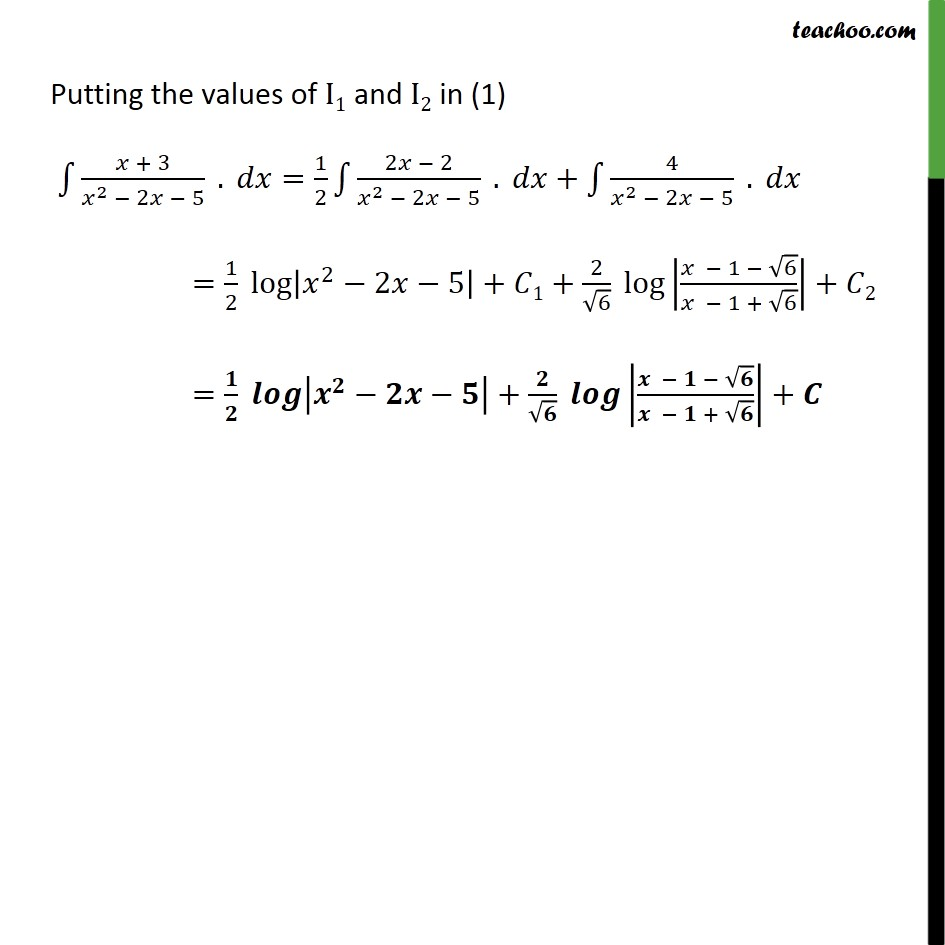1. Chapter 7 Class 12 Integrals
2. Serial order wise
3. Ex 7.4

Transcript

Ex 7.4, 22 Solving I1= 1 2 2 2 2 2 5 . Let 2 2 5= Diff both sides w.r.t.x 2 2 0= = 2 2 Thus, our equation becomes I1= 1 2 2 2 2 2 5 . Putting value of 2 2 5 = and = 2 2 I1= 1 2 2 2 . I1= 1 2 2 2 . 2 2 I1= 1 2 1 . I1= 1 2 log + 1 I1= 1 2 log 2 2 5 + 1 Now, taking I2 I2=4 1 2 2 5 . I2=4 1 2 2 1 5 . I2=4 1 2 2 1 + 1 2 1 2 5 . I2=4 1 1 2 1 2 5 . I2=4 1 1 2 1 5 . I2=4 1 1 2 6 . I2=4 1 1 2 6 2 . I2= 4 2 6 log 1 6 1 + 6 + 2 I2= 2 6 log 1 6 1 + 6 + 2 Putting the values of I1 and I2 in (1) + 3 2 2 5 . = 1 2 2 2 2 2 5 . + 4 2 2 5 . = 1 2 log 2 2 5 + 1+ 2 6 log 1 6 1 + 6 + 2 = + + +

Ex 7.4

Chapter 7 Class 12 Integrals
Serial order wise